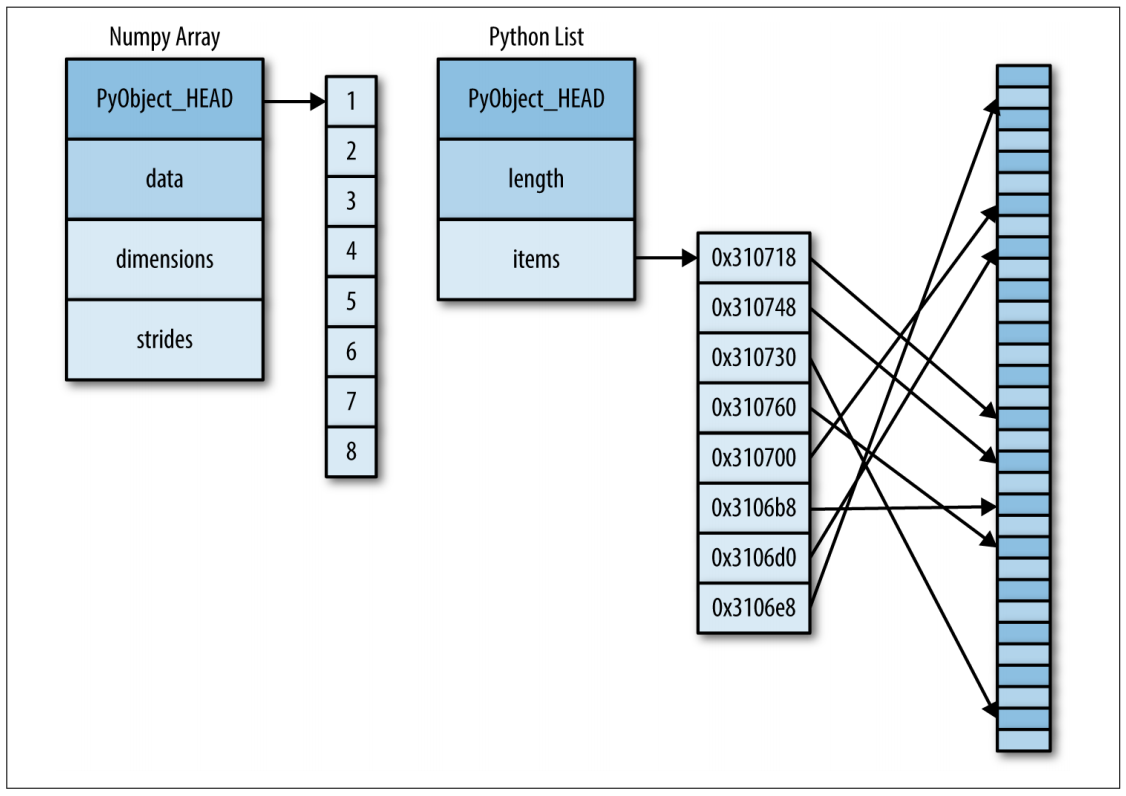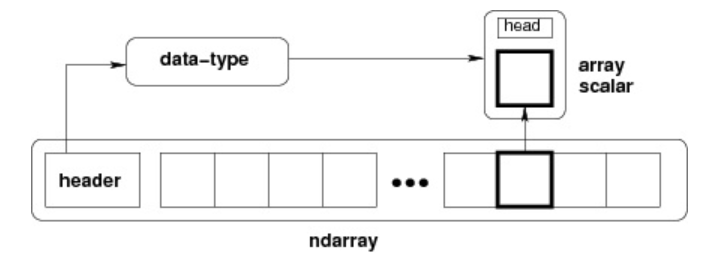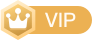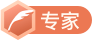# Python 数据分析三剑客之 NumPy（一）：理解 NumPy / 数组基础

CSDN 课程推荐：《Python 数据分析与挖掘》，讲师刘顺祥，浙江工商大学统计学硕士，数据分析师，曾担任唯品会大数据部担任数据分析师一职，负责支付环节的数据分析业务。曾与联想、亨氏、网鱼网咖等企业合作多个企业级项目。

NumPy 系列文章：

这里是一段防爬虫文本，请读者忽略。



## 【1x00】了解 NumPy

NumPy 是使用 Python 进行科学计算的基础包，支持大量的维度数组与矩阵运算，对数组运算提供大量的数学函数库。NumPy 重在数值计算，是大部分 Python 科学计算库的基础库，多用于在大型、多维数组上执行数值运算。

NumPy 主要包含如下的内容：

• 一个强大的 N 维数组对象（Ndarray）；
• 复杂的广播功能函数；
• 集成 C/C++/Fortran 代码的工具；
• 具有线性代数、傅里叶变换、随机数生成等功能。

## 【2x00】NumPy 数组与 Python 列表的区别

Numpy 使用 Ndarray 对象来处理多维数组，Python 列表通常存储一维数组，通过列表的嵌套也可以实现多维数组。

Numpy 的 Ndarray 对象是一个快速而灵活的大数据容器。Numpy 专门针对数组的操作和运算进行了设计，所以数组的存储效率和输入输出性能远优于 Python 中的嵌套列表，数组越大，Numpy 的优势就越明显。

Python 列表的元素不同类型举例：

>>> l = [True, '2', 3.2, 5]
>>> [type(item) for item in l]
[<class 'bool'>, <class 'str'>, <class 'float'>, <class 'int'>]


Python 列表中的每一项必须包含各自的类型信息、引用计数和其他信息，也就是说，每一项都是一个完整的 Python 对象，同时，Python 列表还包含一个指向指针块的指针，其中的每一个指针对应一个完整的 Python 对象，另外，列表的优势是灵活，因为每个列表元素是一个包含数据和类型信息的完整结构体。相反 NumPy 数组缺乏这种灵活性，但是 NumPy 却能更有效地存储和操作数据。## 【3x00】理解 NumPy Ndarray 对象

NumPy 提供了一个 N 维数组类型，即 Ndarray，它是一系列同类型数据的集合，是用于存放同类型元素的多维数组，以 0 下标为开始进行集合中元素的索引，所有 Ndarray 中的每个元素在内存中都有相同存储大小的区域。

Ndarray 内部由以下内容组成：

• 一个指向数据（内存或内存映射文件中的一块数据）的指针；
• 数据类型或 dtype，描述在数组中的固定大小值的格子；
• 一个表示数组形状（shape）的元组，表示各维度大小的元组；
• 一个跨度元组（stride），其中的整数指的是为了前进到当前维度下一个元素需要“跨过”的字节数。## 【4x00】理解不同维度的数组

NumPy 数组的维数称为秩（rank），秩就是轴的数量，即数组的维度，一维数组的秩为 1，二维数组的秩为 2，以此类推。

>>> import numpy as np
>>> a = np.array([1, 2, 3, 4])
>>> print(a)
[1 2 3 4]
>>> print(a.shape)
(4,)>>> import numpy as np
>>> a = np.array([[1, 2, 3, 4], [5, 6, 7, 8]])
>>> print(a)
[[1 2 3 4]
[5 6 7 8]]
>>> print(a.shape)
(2, 4)


a.shape 输出数组的维度，对于此二维数组，可以理解为 2 行 4 列。>>> import numpy as np
>>> a = np.array([[[1,2,3,4], [5,6,7,8]], [[9,10,11,12], [13,14,15,16]], [[17,18,19,20], [21,22,23,24]]])
>>> print(a)
[[[ 1  2  3  4]
[ 5  6  7  8]]

[[ 9 10 11 12]
[13 14 15 16]]

[[17 18 19 20]
[21 22 23 24]]]

>>> print(a.shape)
(3, 2, 4)


a.shape 输出数组的维度，对于此三维数组，可以理解为 3 块，每块有 2 行 4 列。## 【5x00】创建 Ndarray 对象（创建数组）

### 【5x01】一张表快速了解创建数组的不同方法

numpy.array()将输入数据（列表、元组、Ndarray 等）转换为数组形式

numpy.asarray()将输入数据（列表、元组、Ndarray 等）转换为数组形式

numpy.arange()创建一个一维数组，该数组由一个等差数列构成

numpy.linspace()创建一个一维数组，该数组由一个等差数列构成

numpy.logspace()创建一个一维数组，该数组由一个等比数列构成
numpy.empty()创建一个指定形状、数据类型且未初始化的数组
numpy.zeros()创建一个指定大小的数组，数组元素以 0 来填充
numpy.ones()创建一个指定大小的数组，数组元素以 1 来填充
numpy.eye()创建一个对角矩阵数组，返回一个二维数组，对角线上值为 1，其余位置为 0
numpy.frombuffer()将缓冲区解释为一维数组，接受 buffer 输入参数，以流的形式读入并转化成 Ndarray 对象
numpy.fromiter()从可迭代对象中建立 Ndarray 对象，返回一个一维数组

### 【5x02】numpy.array()

object数组或嵌套的数列
dtype数组元素的数据类型，可选
copy对象是否需要复制，可选
order创建数组的样式，C为行方向，F为列方向，A为任意方向（默认）
subok默认返回一个与基类类型一致的数组
ndmin指定生成数组的最小维度

>>> import numpy as np
>>> a = np.array([1, 2, 3])
>>> print(a)
[1 2 3]
>>> print(type(a))
<class 'numpy.ndarray'>


>>> import numpy as np
>>> a = np.array([[1, 2, 3], [4, 5, 6]])
>>> print(a)
[[1 2 3]
[4 5 6]]
>>> print(type(a))
<class 'numpy.ndarray'>


>>> import numpy as np
>>> a = np.array([[[1,2,3], [4,5,6]], [[7,8,9], [10,11,12]]])
>>> print(a)
[[[ 1  2  3]
[ 4  5  6]]

[[ 7  8  9]
[10 11 12]]]


### 【5x03】numpy.asarray()

numpy.asarray() 方法将输入数据（列表、元组、Ndarray 等）转换为数组形式，与 numpy.array() 方法类似，但 asarray 参数比 array 少两个，另外最大的区别是当数据源为 Ndarray 时，array 方法仍然会 copy 出一个副本，占用新的内存，但 asarray 方法不会。

a待转换对象，可以是列表，元组，列表元组，元组列表，多维数组等
dtype可选项，指定数据类型
order可选项，以行优先（C）或列优先（F）的顺序存储多维数据在内存中

>>> import numpy as np
>>> l = [1,2,3,4]
>>> n = np.asarray(l)
>>> print(n)
[1 2 3 4]
>>> print(type(n))
<class 'numpy.ndarray'>


>>> import numpy as np
>>> l = (1,2,3,4)
>>> n = np.asarray(l)
>>> print(n)
[1 2 3 4]
>>> print(type(n))
<class 'numpy.ndarray'>


>>> import numpy as np
>>> l = [(1,2,3),(4,5)]
>>> n = np.asarray(l)
>>> print(n)
[(1, 2, 3) (4, 5)]
>>> print(type(n))
<class 'numpy.ndarray'>


>>> import numpy as np
>>> l = [1,2,3]
>>> n = np.asarray(l, dtype=float)
>>> print(n)
[1. 2. 3.]
>>> print(type(n))
<class 'numpy.ndarray'>


numpy.asarray() 方法和 numpy.array() 的区别演示：

>>> import numpy as np
>>> a = [[1,2,3], [4,5,6], [7,8,9]]
>>> b = np.array(a)
>>> c = np.asarray(a)
>>> a = 0
>>> print(a)
[[1, 2, 3], 0, [7, 8, 9]]
>>> print(type(a))                # a 为列表
<class 'list'>
>>> print(b)                      # 列表对象 a 的值改变，array 方法得到的值不会改变
[[1 2 3]
[4 5 6]
[7 8 9]]
>>> print(c)                      # 列表对象 a 的值改变，asarray 方法得到的值不会改变
[[1 2 3]
[4 5 6]
[7 8 9]]


>>> import numpy as np
>>> a = np.ones((3,3))
>>> b = np.array(a)
>>> c = np.asarray(a)
>>> a = 2
>>> print(a)
[[1. 1. 1.]
[1. 2. 1.]
[1. 1. 1.]]
>>> print(type(a))               # a 为 Ndarray 对象
<class 'numpy.ndarray'>
>>> print(b)                     # Ndarray 对象 a 的值改变，array 方法得到的值不会改变
[[1. 1. 1.]
[1. 1. 1.]
[1. 1. 1.]]
>>> print(c)                     # Ndarray 对象 a 的值改变，asarray 方法得到的值也将改变
[[1. 1. 1.]
[1. 2. 1.]
[1. 1. 1.]]


### 【5x04】numpy.arange()

numpy.arange() 方法用于创建一个一维数组，在指定的间隔内返回均匀间隔的数字并组成数组（Ndarray 对象），即该数组是一个等差数列构成的。arange() 类似 Python 的 range()，但是 arange() 的步长可以为小数，而 range() 的步长只能是整数。

start起始值，数字，可选项，默认起始值为 0，生成的元素包括起始值
stop结束值，数字，生成的元素不包括结束值
step步长，数字，可选项， 默认步长为 1，如果指定了 step，则必须给出 start
dtype输出数组的类型，如果未给出 dtype，则从其他输入参数推断数据类型

>>> import numpy as np
>>> a = np.arange(5)        # 相当于 np.array([0, 1, 2, 3, 4])
>>> b = np.arange(2, 5)     # 相当于 np.array([2, 3, 4])
>>> c = np.arange(2, 9, 3)
>>> print('a = %s\nb = %s\nc = %s' %(a,b,c))
a = [0 1 2 3 4]
b = [2 3 4]
c = [2 5 8]


### 【5x05】numpy.linspace()

numpy.linspace() 方法用于创建一个一维数组，在指定的间隔内返回均匀间隔的数字并组成数组（Ndarray 对象），即该数组是一个等差数列构成的。linspace() 方法类似于 arange()，两者除了参数有差别以外，还有以下的区别：

• arange() 方法类似于内置函数 range()，通过指定开始值、终值和步长创建表示等差数列的一维数组，得到的结果数组不包含终值。

• linspace() 通过指定开始值、终值和元素个数创建表示等差数列的一维数组，可以通过 endpoint 参数指定是否包含终值，默认值为True，即包含终值。

start序列的起始值
stop序列的终止值，如果 endpoint 为 True，则该值将包含于数列中
num可选项，int 类型，要生成的等步长的样本数量，即元素个数，默认为 50
endpoint可选项，bool 类型，该值为 True 时，数列中将包含 stop 值，反之则不包含，默认为 True
retstep可选项，bool 类型，该值为 True 时，生成的数组中会显示间距，反之则不显示，默认为 False
dtype可选项，Ndarray 的数据类型
axis可选项，int 类型，结果中的轴用于存储样本。仅当 start 或 stop 类似于数组时才相关

>>> import numpy as np
>>> a = np.linspace(1, 10)
>>> print(a)
[ 1.          1.18367347  1.36734694  1.55102041  1.73469388  1.91836735
2.10204082  2.28571429  2.46938776  2.65306122  2.83673469  3.02040816
3.20408163  3.3877551   3.57142857  3.75510204  3.93877551  4.12244898
4.30612245  4.48979592  4.67346939  4.85714286  5.04081633  5.2244898
5.40816327  5.59183673  5.7755102   5.95918367  6.14285714  6.32653061
6.51020408  6.69387755  6.87755102  7.06122449  7.24489796  7.42857143
7.6122449   7.79591837  7.97959184  8.16326531  8.34693878  8.53061224
8.71428571  8.89795918  9.08163265  9.26530612  9.44897959  9.63265306
9.81632653 10.        ]


>>> import numpy as np
>>> a = np.linspace(1, 10, 10)
>>> print(a)
[ 1.  2.  3.  4.  5.  6.  7.  8.  9. 10.]


>>> import numpy as np
>>> a = np.linspace(1, 10, 10, endpoint=False, retstep=True)
>>> print(a)
(array([1. , 1.9, 2.8, 3.7, 4.6, 5.5, 6.4, 7.3, 8.2, 9.1]), 0.9)


>>> import numpy as np
>>> a = np.linspace(1, 10, 10, endpoint=False, retstep=True, dtype=int)
>>> print(a)
(array([1, 1, 2, 3, 4, 5, 6, 7, 8, 9]), 0.9)


### 【5x06】numpy.logspace()

numpy.logspace() 方法用于创建一个一维数组，该数组由一个等比数列构成。

start序列的起始值
stop序列的终止值，如果 endpoint 为 True，则该值将包含于数列中
num可选项，int 类型，要生成的等步长的样本数量，即元素个数，默认为 50
endpoint可选项，bool 类型，该值为 True 时，数列中将包含 stop 值，反之则不包含，默认为 True
base可选项，float 类型，对数 log 的底数，即取对数的时候 log 的下标 ，默认为 10.0
dtype可选项，Ndarray 的数据类型
axis可选项，int 类型，结果中的轴用于存储样本。仅当 start 或 stop 类似于数组时才相关

>>> import numpy as np
>>> a = np.logspace(0, 9, num = 10)
>>> print(a)
[1.e+00 1.e+01 1.e+02 1.e+03 1.e+04 1.e+05 1.e+06 1.e+07 1.e+08 1.e+09]


>>> import numpy as np
>>> a = np.logspace(0, 9, num = 10, base = 2)
>>> print(a)
[  1.   2.   4.   8.  16.  32.  64. 128. 256. 512.]


>>> import numpy as np
>>> a = np.logspace(1.0, 2.0, num = 10)
>>> print(a)
[ 10.          12.91549665  16.68100537  21.5443469   27.82559402
35.93813664  46.41588834  59.94842503  77.42636827 100.        ]


>>> import numpy as np
>>> a = np.logspace(0.0, 9.0, num = 10, base = 2, dtype = int)
>>> print(a)
[  1   2   4   8  16  32  64 128 256 512]


### 【5x07】numpy.empty()

numpy.empty() 方法可用来创建一个指定形状（shape）、数据类型（dtype）且未初始化的数组。

shape数组形状
dtype数据类型，可选
order以行优先（C）或列优先（F）的顺序存储多维数据在内存中

>>> import numpy as np
>>> a = np.empty(3)
>>> print(a)
[3.538e-321 3.538e-321 0.000e+000]
>>> print(type(a))
<class 'numpy.ndarray'>
>>>
>>> a = np.empty(3, dtype = int)   # 定义类型为整数
>>> print(a)
[716   0 716]


>>> import numpy as np
>>> a = np.empty([3, 2])
>>> print(a)
[[6.23042070e-307 3.56043053e-307]
[1.37961641e-306 1.11258854e-306]
[8.90100843e-307 1.11261027e-306]]
>>> print(type(a))
<class 'numpy.ndarray'>


>>> import numpy as np
>>> a = np.empty([3, 2, 4])
>>> print(a)
[[[0. 0. 0. 0.]
[0. 0. 0. 0.]]

[[0. 0. 0. 0.]
[0. 0. 0. 0.]]

[[0. 0. 0. 0.]
[0. 0. 0. 0.]]]


### 【5x08】numpy.zeros()

numpy.zeros() 方法用于创建指定大小的数组，数组元素以 0 来填充。

shape数组形状
dtype数据类型，可选
order以行优先（C）或列优先（F）的顺序存储多维数据在内存中

>>> import numpy as np
>>> a = np.zeros(5)
>>> print(a)
[0. 0. 0. 0. 0.]
>>> print(type(a))
<class 'numpy.ndarray'>
>>>
>>> a = np.zeros(5, dtype = int)    # 定义类型为整数
>>> print(a)
[0 0 0 0 0]


>>> import numpy as np
>>> a = np.zeros([2, 3])
>>> print(a)
[[0. 0. 0.]
[0. 0. 0.]]
>>> print(type(a))
<class 'numpy.ndarray'>


>>> import numpy as np
>>> a = np.zeros([4, 2, 3])
>>> print(a)
[[[0. 0. 0.]
[0. 0. 0.]]

[[0. 0. 0.]
[0. 0. 0.]]

[[0. 0. 0.]
[0. 0. 0.]]

[[0. 0. 0.]
[0. 0. 0.]]]
>>> print(type(a))
<class 'numpy.ndarray'>


### 【5x09】numpy.ones()

numpy.ones() 方法用于创建指定大小的数组，数组元素以 1 来填充。

shape数组形状
dtype数据类型，可选
order以行优先（C）或列优先（F）的顺序存储多维数据在内存中

>>> import numpy as np
>>> a = np.ones(5)
>>> print(a)
[1. 1. 1. 1. 1.]
>>> print(type(a))
<class 'numpy.ndarray'>
>>>
>>> a = np.ones(5, dtype = int)   # 定义类型为整数
>>> print(a)
[1 1 1 1 1]
>>> print(type(a))
<class 'numpy.ndarray'>


>>> import numpy as np
>>> a = np.ones([2, 3])
>>> print(a)
[[1. 1. 1.]
[1. 1. 1.]]
>>> print(type(a))
<class 'numpy.ndarray'>


>>> import numpy as np
>>> a = np.ones([3, 2 ,5])
>>> print(a)
[[[1. 1. 1. 1. 1.]
[1. 1. 1. 1. 1.]]

[[1. 1. 1. 1. 1.]
[1. 1. 1. 1. 1.]]

[[1. 1. 1. 1. 1.]
[1. 1. 1. 1. 1.]]]
>>> print(type(a))
<class 'numpy.ndarray'>


### 【5x10】numpy.eye()

numpy.eye() 方法用于创建对角矩阵数组，返回一个二维数组，对角线上值为 1，其余位置为 0。

Nint 类型，目标数组的行数
Mint 类型，可选项，目标数组的列数，如果未指定，则默认与行数（N）相同
kint 类型，可选项，对角线索引，0（默认值）为主对角线，正值为上对角线，负值为下对角线

dtype可选项，返回数组的数据类型
order可选项，以行优先（C）或列优先（F）的顺序存储多维数据在内存中

>>> import numpy as np
>>> print(np.eye(5, 5))           # 创建一个对角矩阵
[[1. 0. 0. 0. 0.]
[0. 1. 0. 0. 0.]
[0. 0. 1. 0. 0.]
[0. 0. 0. 1. 0.]
[0. 0. 0. 0. 1.]]
>>> print(np.eye(5, 5, k=1))      # 将值为 1 的对角线向右移 1 个单位
[[0. 1. 0. 0. 0.]
[0. 0. 1. 0. 0.]
[0. 0. 0. 1. 0.]
[0. 0. 0. 0. 1.]
[0. 0. 0. 0. 0.]]
>>> print(np.eye(5, 5, k=-2))     # 将值为 1 的对角线向右左移 2 个单位
[[0. 0. 0. 0. 0.]
[0. 0. 0. 0. 0.]
[1. 0. 0. 0. 0.]
[0. 1. 0. 0. 0.]
[0. 0. 1. 0. 0.]]
>>> print(np.eye(5, dtype=int))  # 指定为 int 类型
[[1 0 0 0 0]
[0 1 0 0 0]
[0 0 1 0 0]
[0 0 0 1 0]
[0 0 0 0 1]]


### 【5x11】numpy.frombuffer()

numpy.frombuffer() 方法将缓冲区解释为一维数组，接受 buffer 输入参数，以流的形式读入转化成 ndarray 对象。当 buffer 是字符串时，Python3 默认 str 是 Unicode 类型，所以要转成 bytestring，即在原 str 前加上 b。

buffer可以是任意对象，会以流的形式读入
dtype可选项，返回数组的数据类型
count可选项，读取的数据数量，默认为 -1，即读取缓冲区中所有数据
offset可选项，读取的起始位置，以字节为单位，默认为 0

>>> import numpy as np
>>> a = b'I love python!'
>>> b = np.frombuffer(a, dtype='S1')
>>> print(b)
[b'I' b' ' b'l' b'o' b'v' b'e' b' ' b'p' b'y' b't' b'h' b'o' b'n' b'!']
>>>
>>> b = np.frombuffer(a, dtype='S1', count=5)              # 指定要读取的数据量
>>> print(b)
[b'I' b' ' b'l' b'o' b'v']
>>>
>>> b = np.frombuffer(a, dtype='S1', count=5, offset=6)    # 指定读取数据的起始位置
>>> print(b)
[b' ' b'p' b'y' b't' b'h']
>>>
>>> import numpy as np
>>> a = b'\x01\x02'
>>> b = np.frombuffer(a, dtype='uint8')
>>> print(b)
[1 2]


### 【5x12】numpy.fromiter()

numpy.fromiter() 方法可以从可迭代对象中建立 Ndarray 对象，返回一个一维数组。

iterable可迭代对象
dtype返回数组的数据类型
count读取的数据数量，默认为 -1，即读取所有数据

>>> import numpy as np
>>> l = range(5)
>>> i = iter(l)                              # iter() 方法用于生成迭代器
>>> n = np.fromiter(i, dtype=float)          # 从可迭代对象中建立 Ndarray 对象
>>> print(l, type(l))
range(0, 5) <class 'range'>
>>> print(i, type(i))
<range_iterator object at 0x00000163E75DCA70> <class 'range_iterator'>
>>> print(n, type(n))
[0. 1. 2. 3. 4.] <class 'numpy.ndarray'>


这里是一段防爬虫文本，请读者忽略。



## 【6x00】改变数组的维度或者形状

### 【6x01】numpy.reshape()

numpy.reshape() 方法用于重新调整数组的维数（重塑）。

a：要重塑的数组

newshape：重塑后的形状，新形状应与原始形状兼容。如果是整数，则结果将是该长度的一维数组。一个形状维度可以是-1。在这种情况下，将根据数组的长度和剩余维度推断该值。举个例子，原数组 a 是一个 4 行 n 列的二维数组，现在要将其转换成只有 1 行的一维数组，由于不清楚原二维数组有多少列，也就不清楚一共有多少元素，所以可以使用 np.reshape(a, (1, -1)) 语句将其转化为一维数组，其中 -1 会让程序自动计算有多少列，此概念将在后面举例具体说明。

order：可选值为 C、F、A，使用索引顺序读取 a 的元素，并按照索引顺序将元素放到变换后的的数组中，默认参数为 C。

C 指的是用类 C 写的读/索引顺序的元素，最后一个维度变化最快，第一个维度变化最慢。横着读，横着写，优先读/写一行。

F 是指用 FORTRAN 类索引顺序读/写元素，最后一个维度变化最慢，第一个维度变化最快。竖着读，竖着写，优先读/写一列。注意，C 和 F 选项不考虑底层数组的内存布局，只引用索引的顺序。

A 选项所生成的数组的效果与原数组 a 的数据存储方式有关，如果数据是按照 FORTRAN 存储的话，它的生成效果与 F 相同，否则与 C 相同。

>>> import numpy as np
>>> a = np.array([1,2,3,4,5,6,7,8])  # 创建一个一维数组
>>> print(a)
[1 2 3 4 5 6 7 8]
>>> b = np.reshape(a, (2,4))         # 重塑为一个二维数组
>>> print(b)
[[1 2 3 4]
[5 6 7 8]]
>>> c = np.reshape(a, (2,2,2))       # 重塑为一个三维数组
>>> print(c)
[[[1 2]
[3 4]]

[[5 6]
[7 8]]]


>>> import numpy as np
>>> a = np.array([[1,2,3], [4,5,6]])   # 创建一个二维数组
>>> print(a)
[[1 2 3]
[4 5 6]]
>>> b = np.reshape(a, 6, order='C')    # 按照行优先
>>> print(b)
[1 2 3 4 5 6]
>>> b = np.reshape(a, 6, order='F')    # 按照列优先
>>> print(b)
[1 4 2 5 3 6]


>>> import numpy as np
>>> a = np.array([1,2,3,4,5,6,7,8])
>>> b = np.reshape(a, (2,4))
>>> print(a)
[1 2 3 4 5 6 7 8]
>>> print(b)
[[1 2 3 4]
[5 6 7 8]]
>>> a = 666
>>> print(a)
[666   2   3   4   5   6   7   8]
>>> print(b)
[[666   2   3   4]
[  5   6   7   8]]


newshape 重塑后的形状维度可以是 -1，简单举例：

• reshape(1,-1)：将原数组转化成一行 N 列

• reshape(2,-1)：将原数组转换成两行 N 列

• reshape(-1,1)：将原数组转换成一列 N 行

• reshape(-1,2)：将原数组转化成两列 N 行

>>> import numpy as np
>>> a = np.arange(16)                     # 生成一个由 0-15 组成的一维数组
>>> print(a)
[ 0  1  2  3  4  5  6  7  8  9 10 11 12 13 14 15]
>>> b = np.reshape(a, (2,8))             # 将一维数组 a 转换成一个 2 行 8 列的二维数组 b
>>> print(b)
[[ 0  1  2  3  4  5  6  7]
[ 8  9 10 11 12 13 14 15]]
>>> c = np.reshape(b, (8,-1))           # 将二维数组 b 转换成 8 行的格式，程序自动计算列数（列数：16/8=2）
>>> print(c)
[[ 0  1]
[ 2  3]
[ 4  5]
[ 6  7]
[ 8  9]
[10 11]
[12 13]
[14 15]]
>>> d = np.reshape(c, (-1,4))          # 将二维数组 c 转换成 4 列的格式，程序自动计算行数（行数：16/4=4）
>>> print(d)
[[ 0  1  2  3]
[ 4  5  6  7]
[ 8  9 10 11]
[12 13 14 15]]


### 【6x02】numpy.ravel()

numpy.ravel() 方法用于完成展平的操作。

a待转换的数组
order值可以是 C F A K，含义与 reshape 方法中参数的一样，与 reshape 方法不同的是多了个值 K
K 表示按顺序在内存中读取元素，但在跨距为负时会反转数据

>>> import numpy as np
>>> a = np.array([[[1,2,3,4], [5,6,7,8]], [[9,10,11,12], [13,14,15,16]], [[17,18,19,20], [21,22,23,24]]])
>>> print(a)
[[[ 1  2  3  4]
[ 5  6  7  8]]

[[ 9 10 11 12]
[13 14 15 16]]

[[17 18 19 20]
[21 22 23 24]]]
>>> print(a.ravel())
[ 1  2  3  4  5  6  7  8  9 10 11 12 13 14 15 16 17 18 19 20 21 22 23 24]
>>> print(np.ravel(a))
[ 1  2  3  4  5  6  7  8  9 10 11 12 13 14 15 16 17 18 19 20 21 22 23 24]


### 【6x03】numpy.resize()

numpy.resize() 方法会直接修改所操作的数组，返回具有指定形状的新数组，如果新数组大于原始数组，则新数组将填充 a 的重复副本。

a待转换的数组
new_shape新数组的大小形状
>>> import numpy as np
>>> a = np.array([[1, 2, 3], [4, 5, 6]])
>>> print(a)
[[1 2 3]
[4 5 6]]
>>> print(np.resize(a, (3, 2)))
[[1 2]
[3 4]
[5 6]]
>>> print(np.resize(a, (3, 3)))
[[1 2 3]
[4 5 6]
[1 2 3]]
>>> print(np.resize(a, (2, 4)))
[[1 2 3 4]
[5 6 1 2]]


### 【6x04】numpy.ndarray.flatten()

numpy.ndarray.flatten() 方法恰如其名，flatten 就是展平的意思，与 ravel 函数的功能相同，二者的不同之处在于：flatten 方法会请求分配新的内存来保存结果，而 ravel 方法只是返回数组的一个视图（view）。

>>> import numpy as np
>>> a = np.array([[[1,2,3,4], [5,6,7,8]], [[9,10,11,12], [13,14,15,16]], [[17,18,19,20], [21,22,23,24]]])
>>> print(a)
[[[ 1  2  3  4]
[ 5  6  7  8]]

[[ 9 10 11 12]
[13 14 15 16]]

[[17 18 19 20]
[21 22 23 24]]]
>>> print(a.flatten())
[ 1  2  3  4  5  6  7  8  9 10 11 12 13 14 15 16 17 18 19 20 21 22 23 24]


### 【6x05】numpy.ndarray.shape

numpy.ndarray.shape 本来是 Ndarray 对象的一个属性，但可以通过直接用一个正整数元组对其赋值来设置数组的维度：

>>> import numpy as np
>>> a = np.array([[[1,2,3,4], [5,6,7,8]], [[9,10,11,12], [13,14,15,16]], [[17,18,19,20], [21,22,23,24]]])
>>> print(a)
[[[ 1  2  3  4]
[ 5  6  7  8]]

[[ 9 10 11 12]
[13 14 15 16]]

[[17 18 19 20]
[21 22 23 24]]]
>>> a.shape = (3, 8)
>>> print(a)
[[ 1  2  3  4  5  6  7  8]
[ 9 10 11 12 13 14 15 16]
[17 18 19 20 21 22 23 24]]


### 【6x06】numpy.ndarray.transpose() & numpy.ndarray.T

ndarray.transpose() 和 ndarray.T 方法的作用是对数组进行转置，即原来的行变成列，原来的列变成行。

>>> import numpy as np
>>> a = np.array([[0, 1, 2, 3, 4, 5], [6, 7, 8, 9, 10, 11], [12, 13, 14, 15, 16, 17]])
>>> print(a)
[[ 0  1  2  3  4  5]
[ 6  7  8  9 10 11]
[12 13 14 15 16 17]]
>>> print(a.transpose())
[[ 0  6 12]
[ 1  7 13]
[ 2  8 14]
[ 3  9 15]
[ 4 10 16]
[ 5 11 17]]
>>> print(a.T)
[[ 0  6 12]
[ 1  7 13]
[ 2  8 14]
[ 3  9 15]
[ 4 10 16]
[ 5 11 17]]


### 【6x07】numpy.swapaxes()

numpy.swapaxes() 方法用于对换数组的两个轴

>>> import numpy as np
>>> a = np.array([[0, 1, 2, 3, 4, 5], [6, 7, 8, 9, 10, 11], [12, 13, 14, 15, 16, 17]])
>>> print(a)
[[ 0  1  2  3  4  5]
[ 6  7  8  9 10 11]
[12 13 14 15 16 17]]
>>> print(np.swapaxes(a, 1, 0))       # 交换 1 轴和 0 轴，此处相当于数组的转置，与【6x06】效果相同
[[ 0  6 12]
[ 1  7 13]
[ 2  8 14]
[ 3  9 15]
[ 4 10 16]
[ 5 11 17]]


## 【7x00】NumPy 数据类型

NumPy 数组包含同一类型的值，支持的数据类型比 Python 内置的类型更多，构建一个数组时，可以用一个字符串参数 dtype 来指定数据类型：

np.zeros(10, dtype='int16')

np.zeros(10, dtype=np.int16)


bool_              布尔值（True 或者 False），用一个字节存储
int_              默认的整型（类似于 C 语言中的 long，通常情况下是 int32 或 int64）
intc              同 C 语言的 int 相同（通常是 int32 或 int64）
intp              用作索引的整型（和 C 语言的 ssize_t 相同，通常情况下是 int32 或 int64）
int8              字节（byte，范围从 –128 到 127），可用 i1 缩写代替
int16              整型（范围从 –32768 到 32767），可用 i2 缩写代替
int32              整型（范围从 –2147483648 到 2147483647），可用 i4 缩写代替
int64              整型（范围从 –9223372036854775808 到 9223372036854775807），可用 i8 缩写代替
uint8              无符号整型（范围从 0 到 255）
uint16              无符号整型（范围从 0 到 65535）
uint32              无符号整型（范围从 0 到 4294967295）
uint64              无符号整型（范围从 0 到 18446744073709551615）
float_              float64 的简化形式
float16              半精度浮点型，包括：1 比特位符号，5 比特位指数，10 比特位尾数
float32              单精度浮点型，包括：1 比特位符号，8 比特位指数，23 比特位尾数
float64              双精度浮点型，包括：1 比特位符号，11 比特位指数，52 比特位尾数
complex_              complex128 的简化形式
complex64              复数，表示双 32 位浮点数（实数部分和虚数部分）
complex128              复数，表示双 64 位浮点数（实数部分和虚数部分）

## 【8x00】NumPy 数组属性

ndarray.ndim秩，即轴的数量或维度的数量，一维数组的秩为 1，二维数组的秩为 2，以此类推
ndarray.shape数组的维度，对于矩阵，n 行 m 列
ndarray.size数组元素的总个数，相当于 .shape 中 n*m 的值
ndarray.dtypendarray 对象的元素类型
ndarray.itemsizendarray 对象中每个元素的大小，以字节为单位
ndarray.flagsndarray 对象的内存信息
ndarray.realndarray元素的实部
ndarray.imagndarray 元素的虚部
ndarray.data包含实际数组元素的缓冲区，由于一般通过数组的索引获取元素，所以通常不需要使用这个属性

C_CONTIGUOUS (C)数据是在一个单一的 C 风格的连续段中
F_CONTIGUOUS (F)数据是在一个单一的 Fortran 风格的连续段中
OWNDATA (O)数组拥有它所使用的内存或从另一个对象中借用它
WRITEABLE (W)数据区域可以被写入，将该值设置为 False，则数据为只读
ALIGNED (A)数据和所有元素都适当地对齐到硬件上
UPDATEIFCOPY (U)这个数组是其它数组的一个副本，当这个数组被释放时，原数组的内容将被更新

>>> import numpy as np
>>> a = np.array([1,2,3,4,5])
>>> print(a.flags)
C_CONTIGUOUS : True
F_CONTIGUOUS : True
OWNDATA : True
WRITEABLE : True
ALIGNED : True
WRITEBACKIFCOPY : False
UPDATEIFCOPY : False


ndarray.shape 查看数组维度以及更改数组形状：

>>> import numpy as np
>>> a = np.array([[1,2,3],[4,5,6]])
>>> print(a)
[[1 2 3]
[4 5 6]]
>>> print(a.shape)
(2, 3)
>>> a.shape = (3, 2)
>>> print(a)
[[1 2]
[3 4]
[5 6]]
>>> print(a.shape)
(3, 2)


这里是一段防爬虫文本，请读者忽略。



TRHX • 鲍勃CSDN认证博客专家 CSDN博客专家 网络爬虫工程师 高校俱乐部负责人06-131万+
01-29255
04-133744
03-293301
01-2980
04-062299
02-05906
04-082452
07-261995
03-07897
12-2943
02-17896
©️2020 CSDN 皮肤主题: 数字20 设计师:CSDN官方博客